# 划分问题：三维匹配问题、图着色问题

## 6 划分问题

【三维匹配问题】

### 6.2 三维匹配问题是NP完全的

【3-SAT\leq_p三维匹配证明】

A_i={a_{i,1},a_{i,2},…,a_{i,2k}}
B_i={b_{i,1},b_{i,2},…,b_{i,2k}}
t_{i,j}={(a_{i,j},a_{i,j+1},b_{i,j}}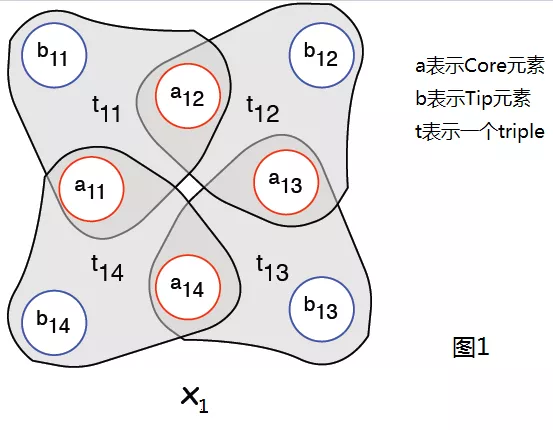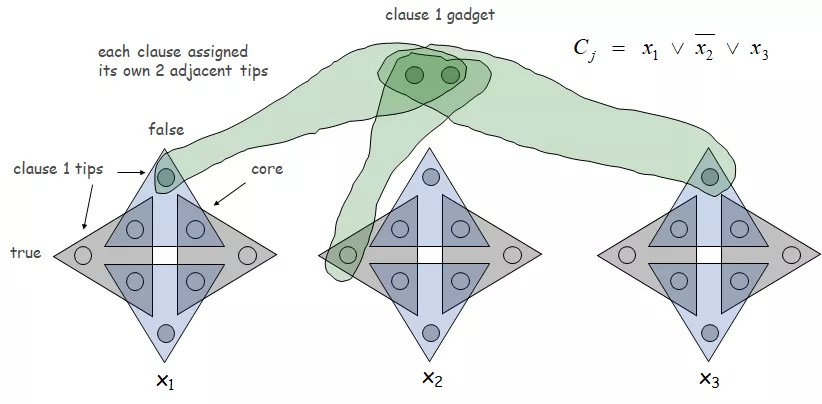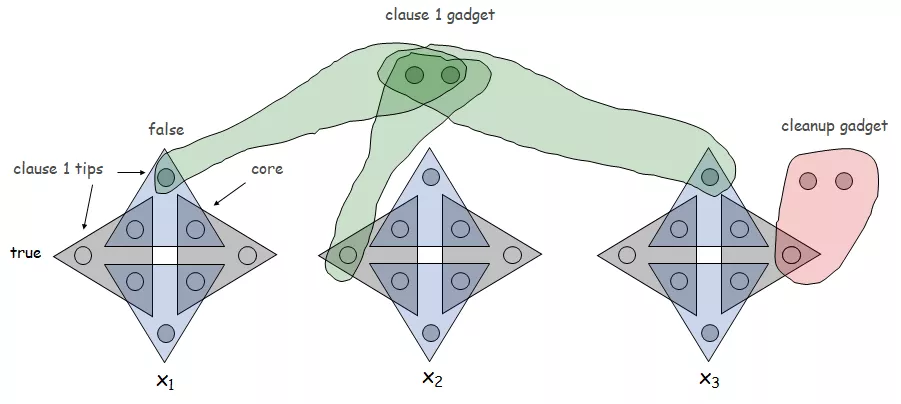X={a_{i,j}(j是偶数)} \vee{p_j} \vee {q_j} 。
Y={a_{i,j}(j是奇数)} \vee{p_j’} \vee {q_j’} 。
Z={b_{i,j}} 。
T={所有构造出来的三元组}。

【对于每一个3-SAT实例】

T={变量中选择的三元组}\vee{子句中选择的三元组}\vee{cleanup中的三元组}
【对于一个三维匹配实例】

【图着色问题】

### 6.4 三着色问题是NP完全的

【3-SAT\leq_p三着色】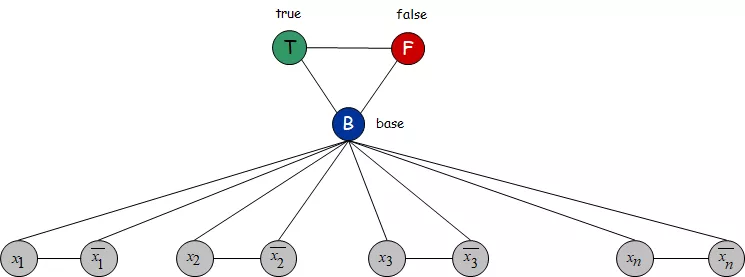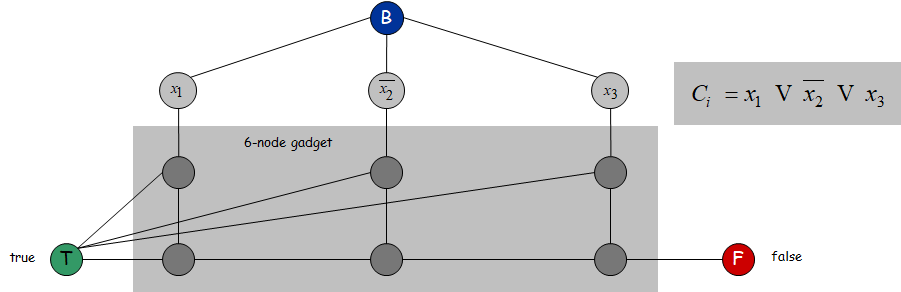​图13 附加的子图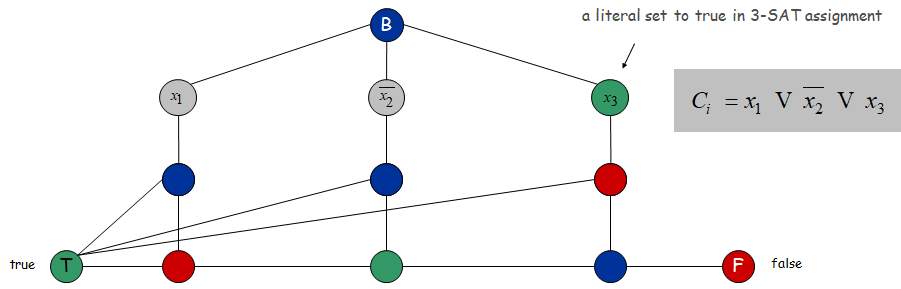0